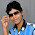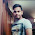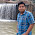## Monday, November 09, 2015

### Understanding SI and CI by Solving Questions Video(17 QUESTIONS)

In this video an attempt to revise you the concepts of Simple Interest and Compound Interest has been done and ways of solving their problems have also been discussed. I hope you all will like the video. Do remember to leave your views in the comment section below. I would be more than happy to read them as it would help me assess my efforts. HAPPY STUDIES !!!!

Question 1. Seema lent part of the Rs. 10000 that she had to a friend at 8 % Simple Interest for four years. She invested the remaining amount at 12 % Simple Interest. The total income from interest after four years was Rs. 3800. What was the amount that Seema lent to her friend ??

Question 2. The rate of interest for the first two years is 3 percent per annum. For the next three years it is 8 % per annum and for the period beyond 5 years it is 10 % per annum. If a man gets Rs. 1520 as simple interest for 6 years, how much did he deposit ??

Question 3. Simple Interest on a certain sum is 9/16 of the sum. Find the rate percent and the time in years, if both of them are equal.

Question 4. If the difference between the Compound Interest and Simple Interest at the end of two years for the same principal is Rs. 100, what is the principal amount ? Rate is 5 percent per annum in both the cases.

Question 5. An amount doubles in seven years when invested under Compound Interest at a certain rate. In how many years will the amount become eight times of the original amount at the same rate ????

Question 6. Ram and Shyam invested some money at 6 % and 7 % per annum Simple Interest respectively. At the end of two years they found that together they received Rs. 354 as interest. One fourth of Ram's initial investment is equal to one fifth of the money invested by Shyam. Find the total money that has been invested ??

Question 7. The Compound Interest on a certain sum of money for two years at 10 % per annum is Rs. 420. The Simple Interest on the same sum at the same rate and for the same time will be

Question 8. A sum of money is kept in a bank at 8 % interest compounded annually. Had the interest been 10 % compounded semi annually, the amount after one year would have been Rs. 225 more. What was the principal amount kept in the bank ??

Question 9. A man invested Rs. 1000 at the rate of 15 % per annum at Simple Interest. He withdraws the final amount after T years. He keeps half of the withdrawn amount with him and invests the remaining. This invested amount kept on reducing at simple interest rate of 12.5 % per annum for a period of 6 years. If the aggregate sum with the man after T+6 years is Rs. 1000, then find the value of T ?

Question 10. A man borrowed Rs. 30000 from a bank at compound interest, interest being compounded annually. At the end of every year he paid Rs. 9000. At the end of the third year, he wanted to clear the loan. How much should he pay to clear the loan ?

Question 11. Mahinder borrowed a certain sum of money at certain rate of Simple Interest. If, instead the amount was borrowed at Compound Interest, the interest for the first two years increases by Rs. 100, while the total interest for the first three years increased by Rs. 305. Find the sum.

Question 12. A sum of money invested at Simple Interest amounts to Rs. 10,240 at the end of three years. If the interest at the end of five years is the same as the sum invested, then the interest at the end of first four years is

Question 13. A sum of money under Compound Interest, interest being compounded half yearly, doubles itself in three years. In how many years will it become 32 times itself ??

Question 14. A sum of money invested at Simple Interest doubles itself in eight years. In how many years will it become 16 times itself at double this rate of Simple Interest ??

Question 15. The difference between compound interest compounded annually at 10 % per annum and simple interest at 12 % per annum on a certain sum of money for two years is Rs. 2640. Find the sum

Question 16. Sanjay borrowed Rs. 75,000 from two banks at compound interest, compounded annually. One bank charges interest at the rate of 15 % per annum and the other bank at 20 % per annum. If at the end of the year, Sanjay paid Rs. 12,000 as the total interest to the two banks, how much did he borrow from the second bank ??

Question 17. Hina borrowed a certain sum from a money lender under compound interest, compounded annually. If the interest for the third year is four times the interest for the first year, then what is the per annum rate of interest ?

1.Hi Sir,
This video is not working.

2.Can you upload the video again.

3.Video not available. Can you please upload again.

4.sir pls upload the video plsss....

5.what happened to this video :(

6.Sir upload the video plz

Add a Comment or Query